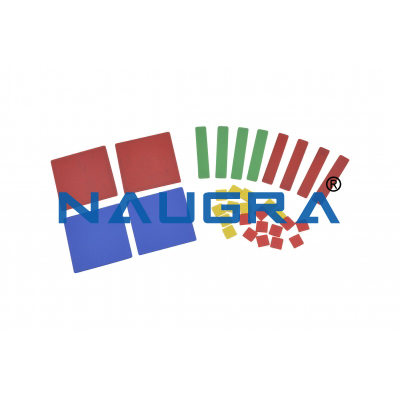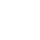• Enquiry

# Algebra TilesProduct Name: Algebra Tiles
Product Code: Mathlab0002

Description and Specification for Lab Tenders

Algebra Tiles.
Many students struggle with factoring polynomials. Polynomials are a number of mathematical terms that can be added, subtracted and multiplied.
For example, 5x2+3x+9 would be a typical polynomial.
Algebra tiles are manipulative devices that students can use to work math problems.
These Algebra tiles come in the shapes of squares and rectangles.
Using algebra tiles in teaching polynomials allows students to practice working with polynomials with a hands-on approach.
The tiles provide a visual representation of the problem that then can be worked by manipulating the tiles.
Algebra tiles come with three types of tiles in two colors each, typically red for negative tiles and blue for positive tiles

## Related Products

Other Related Teaching Lab Products, Scientific Instruments and Educational Equipments like Algebra Tiles. . .